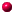Geometry and Asymptotics If you are interested in the field of non-linear differential equations and want to know how to describe their solutions, you need to develop a new toolbox of mathematical methods. Some of these may be familiar to you. For example, there are classical techniques that allow you to find explicit solutions of non-linear ODEs like where $$y=y(t)$$. This special case of a Riccati equation can be solved by separation of variables. There are two types of solutions: (The second case can be thought of as the limit $$t_0\to\infty$$ of the first.) However, for most non-linear ODEs, there is no method of finding an explicit solution. In fact, for equations such as the Painlevé equations, it is known that the general solution cannot be expressed in terms of classical (i.e., earlier known) functions. These notes aim to provide another way of describing the behaviours of such solutions, starting from the point of view of geometry. To find out more, you will need to read the pages below. CONTENTS: 1 Algebraic Curves 1.1 Motivation 1.2 Local Asymptotics of the first Painlevé equation updated 13Jul12 1.3 Local Asymptotics of a discrete Painlevé equation updated 13Jul12 1.4 Projective Geometryupdated 13Jul12Home Page The contents will be expanded gradually. If there is something you are dying to find out, email me. Last modified: 17 July 2012 by Nalini Joshi